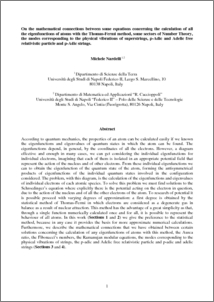# On the mathematical connections between some equations concerning the calculation of all the eigenfunctions of atoms with the Thomas-Fermi method, some sectors of Number Theory, the modes corresponding to the physical vibrations of superstrings, p-Adic and Adelic free relativistic particle and p-Adic strings.

Nardelli, Michele (2009) On the mathematical connections between some equations concerning the calculation of all the eigenfunctions of atoms with the Thomas-Fermi method, some sectors of Number Theory, the modes corresponding to the physical vibrations of superstrings, p-Adic and Adelic free relativistic particle and p-Adic strings. Dip.Sc.Terra-Dip.Matem.Unina. (Unpublished)Preview
PDF
NardFam1.pdfView Item